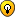Simple lights effects

Share your awesome Ringo behaviors here!
AngoLito
Posts: 5
Joined: Sat May 21, 2016 8:09 pm

Simple lights effects

Hi!

I'm starting to "play" with my Ringo; I want to share this simple sketch of light effectsFinally I've copied part of Behavior 5 (color wheel) to change animation's colors.

Is very elemental... but in a dark room Ringo makes a nice effect, specially "moving light"To test, simply open Ringo_Base_Sketch_Rev06_01, or download from PlumGeek GitHub here
and replace all code in main sketch ("Ringo_base_sketch...") for next code:

Code: Select all

/*
First steps with Leds
Program based on Plumggek guide examples
AngoLito - May 2016 - Luanda

Efects:
- Moving light
- Flash eyes
- Dimmer down face Led

Used Behavior 5 sketch (color Wheel) to change color trought Gyro sensor
*/

//Be sure on QUOTATION MARKS pasting code from different Arduino editor fonts can give error
#include "RingoHardware.h"

byte i;            //general purpouse
byte j=255;     //Bright Led level
byte k;           //general purpouse
byte num_pixel[] = {0,3,4,2,1}; //used to lights Led in order on moving light effect
byte red;       //Red colour value
byte green;   //Green colour value
byte blue;     //Blue colour value
unsigned long tiempo;    //Used on <retardo> routine to make delay

int presentDirection;
int hue;
int hueOpposite;
double r=0;        //Used in setLedColorHSV routine and dimmer mode (PreLoaded Behavior case 5)
double g=0;
double b=0;

void setup(){
HardwareBegin();          //initialize Ringo's brain to work with his circuitry
PlayStartChirp();           //Play startup chirp and blink eyes
SwitchMotorsToSerial(); //Call "SwitchMotorsToSerial()" before using Serial.print functions as motors & serial share a line
RestartTimer();
}

void loop(){

// moving lights
for (k=0; k<19; k++) {
for (i=0; i<5; i++) {
SetPixelRGB(num_pixel[i],red,green,blue);  //set Led
if (num_pixel[i]==4) {
SetPixelRGB( 5, red, green, blue);   //both eyes
}
retardo(9);
OffPixels();
retardo(75);  //Calls subroutine <retardo> for delay and colour adjust
}
}

// dimmer down face lights
for (i=0; i<3; i++){
for (j=0; j<255; j++) {                 // dimmer lights from 0 to 255
SetPixelRGB(1,red,green,blue); // lights two down face Leds
SetPixelRGB(2,red,green,blue);
retardo(4);
}
for (j=255; j>0; j--) {                // dimmer lights from 255 to 0
SetPixelRGB(1,red,green,blue);
SetPixelRGB(2,red,green,blue);
retardo(4);
}
}

// flashing eyes
j=255;                        //Set maximun bright for Led
for (k=0; k<11; k++) {
for (i=0; i<5; i++){
OnEyes(red,green,blue);
retardo(5);
OffPixels();
retardo(65);
}
retardo(375);
}
} // end of loop() function

// In delay time adjust color calling ledwheel()
void retardo(int a) {
tiempo = millis();
while (millis()<(tiempo+a)) {
ledwheel();
}
}

// Calculate color_from Plumgeek PreLoaded Behaviors
void ledwheel() {
SimpleGyroNavigation();                          //just does gyroscope (not accelerometer)
presentDirection = abs(GetDegrees());     //gets the present heading in degrees, result is between -180 ~ +180
presentDirection = presentDirection%360;  //If it's been turned multiple times, this divides the large degree number back into a 360 base
hue = presentDirection;                      //Eduardo's function conveniently takes the same 360 degree value we have related to the gyro
hueOpposite = (hue + 180)%360;         //Get the opposite color on the wheel
//SwitchButtonToPixels();                 //User button not used in this sketch
setLedColorHSV(hue,1,1);                 //Calculate the RGB values based on hue
}

void setLedColorHSV(int h, double s, double v) {
//this is the algorithm to convert from RGB to HSV
double hf=h/60.0;
int i=(int)floor(h/60.0);
//int i=(int)(h/60.0);       //just testing
double f = h/60.0 - i;
double pv = v * (1 - s);
double qv = v * (1 - s*f);
double tv = v * (1 - s * (1 - f));

switch (i)
{
case 0: //rojo dominante
r = v;
g = tv;
b = pv;
break;
case 1: //verde
r = qv;
g = v;
b = pv;
break;
case 2:
r = pv;
g = v;
b = tv;
break;
case 3: //azul
r = pv;
g = qv;
b = v;
break;
case 4:
r = tv;
g = pv;
b = v;
break;
case 5: //rojo
r = v;
g = pv;
b = qv;
break;
}

//set each component to a integer value between 0 and 255
red=constrain((int)j*r,0,255);            //using byte j is a little modification from original code to allows Led dimmer
green=constrain((int)j*g,0,255);
blue=constrain((int)j*b,0,255);

} // Ends setLedColorHSV routine

I hope you enyoi it!
I hear and I forget. I see and I remember. I do and I understand (Confucius)

Who is online

Users browsing this forum: No registered users and 1 guest